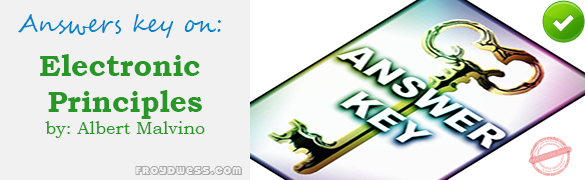# Malvino: MCQ in Diode Theory – Answers

(Last Updated On: February 7, 2020)Below are the answers key for Multiple Choice Questions in Chapter 3: Diode Theory from the book Electronic Principles 7th Edition by Albert Malvino. Make sure to familiarize each and every questions to increase the chance of passing the ECE Board Exam.

1. b. Linear

2. b. Linear

3. c. Nonlinear

4. d. Reverse

5. a. Forward

6. b. Barrier potential

7. c. Surface-leakage current

8. c. 0.7 V

9. a. 0

10. a. 0

11. b. 0.23 ohm

12. b. Vertical

13. a. Troubleshooting

14. d. All of the above

15. a. Load resistance is low

16. c. 15 mA

17. b. 14.3 mA

18. b. 14.3 mA

19. a. 0

20. b. 15 V

21. b. An open diode